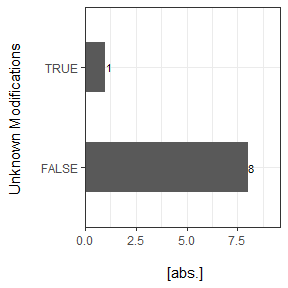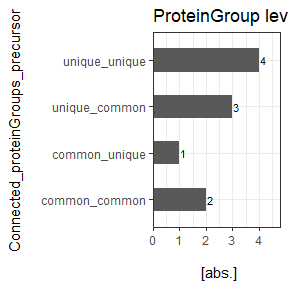# Introduction

This vignette is a workflow template including import, conversion to a standard format and highlighting inter-software proteinGroup denotation differences with flowTraceR.

library(flowTraceR)
library(magrittr)
library(dplyr)
library(tidyr)
library(stringr)
library(tibble)
library(ggplot2)
library(data.table)
library(kableExtra)

# Import

## Import your data

Importing the output files from each software can be easily performed with data.table::fread().

diann <- data.table::fread("DIRECTORY/dia-nn_file.tsv")
pd_psm <- data.table::fread("DIRECTORY/pd_PSMs.txt")

## Examples

Some examples are provided to explore the workflow.

#DIA-NN
diann <- flowTraceR::get_example("DIA-NN")

#Spectronaut
spectronaut <- flowTraceR::get_example("Spectronaut")

#MaxQuant
mq_evidence <- flowTraceR::get_example("MaxQuant")[["evidence"]]

mq_proteinGroups <- flowTraceR::get_example("MaxQuant")[["proteinGroups"]]

#PD
pd_psm <- flowTraceR::get_example("PD")

# Conversion to standardized format

The input data can be converted to a standardized output format on precursor, modified peptide and proteingroup level. The generated columns with flowTraceR are appendend to the submitted data without any filtering performed. The generated columns are denoted with the prefix traceR. Note that only the modifications UniMod:35 (Oxidation) and UniMod:4 (Carbamidomethyl) are supported by flowTraceR. A column with the appendix unknownMods is generated to potentially filter modifications which are not supported: if TRUE, an unknown modification is detected.

## Precursor

For converting the precursor level use convert_precursor().

diann_precursor_converted <- convert_precursor(input_df = diann, software = "DIA-NN")
spectronaut_precursor_converted <- convert_precursor(input_df = spectronaut, software = "Spectronaut")
mq_precursor_converted <- convert_precursor(input_df = mq_evidence, software = "MaxQuant")
pd_precursor_converted <- convert_precursor(input_df = pd_psm, software = "PD")

## Modified Peptides

For converting the modified peptide level use convert_modified_peptides().

diann_peptides_converted <- convert_modified_peptides(input_df = diann, software = "DIA-NN")
spectronaut_peptides_converted <- convert_modified_peptides(input_df = spectronaut, software = "Spectronaut")
mq_peptides_converted <- convert_modified_peptides(input_df = mq_evidence, software = "MaxQuant")
pd_peptides_converted <- convert_modified_peptides(input_df = pd_psm, software = "PD")

## ProteinGroups

For converting the proteinGroup level use convert_proteingroups().

diann_proteinGroups_converted <- convert_proteingroups(input_df = diann, software = "DIA-NN")
spectronaut_proteinGroups_converted <- convert_proteingroups(input_df = spectronaut, software = "Spectronaut")
mq_proteinGroups_converted <- convert_proteingroups(input_df = mq_proteinGroups, software = "MaxQuant")
pd_proteinGroups_converted <- convert_proteingroups(input_df = pd_psm, software = "PD")

## All Levels

For converting precursor, modified peptide and proteingroup level at once use convert_all_levels().

diann_all_converted <- convert_all_levels(input_df = diann, software = "DIA-NN")
spectronaut_all_converted <- convert_all_levels(input_df = spectronaut, software = "Spectronaut")
mq_all_converted <- convert_all_levels(input_df = mq_evidence, input_MQ_pg = mq_proteinGroups, software = "MaxQuant")
pd_all_converted <- convert_all_levels(input_df = pd_psm, software = "PD")

## Analyzing Conversion

Since only the modifications UniMod:35 (Oxidation) and UniMod:4 (Carbamidomethyl) are currently supported, flowTraceR provides functions to analyze the conversion and shows how much unknown modifications are present in the dataset with analyze_unknown_mods().

#For one software example - equivalent for others.

#Proteome Discoverer
#Reports
pd_precursor_report_unknown_mods <- analyze_unknown_mods(input_df = pd_precursor_converted, level = "precursor", plot = FALSE)
pd_peptides_report_unknown_mods <- analyze_unknown_mods(input_df = pd_peptides_converted, level = "modified_peptides", plot = FALSE)

#Plots
pd_precursor_plot_unknown_mods <- analyze_unknown_mods(input_df = pd_precursor_converted, level = "precursor", plot = TRUE, plot_characteristic = "absolute")
pd_peptides_plot_unknown_mods <- analyze_unknown_mods(input_df = pd_peptides_converted, level = "modified_peptides", plot = TRUE, plot_characteristic = "relative")

### Example precursor level

kableExtra::kable(pd_precursor_report_unknown_mods)
Unknown_Modifications absolute_count relative_count
FALSE 8 88.9
TRUE 1 11.1

pd_precursor_plot_unknown_mods# Tracing inter-software differences

For binary software comparisons flowTraceR allows to trace inter-software differences based on the standardized flowTraceR format. Each identification is classified as common - identified in both analyses or as unique - specific to one analysis.

## For each individual level

#Binary Comparison - DIA-NN vs. Spectronaut

#ProteinGroup level
traced_proteinGroups <- trace_level(input_df1 = diann_all_converted , input_df2 = spectronaut_all_converted, analysis_name1 = "DIA-NN", analysis_name2 = "Spectronaut", level = "proteinGroups", filter_unknown_mods = TRUE)

#Peptide level
traced_peptides <- trace_level(input_df1 = diann_all_converted, input_df2 = spectronaut_all_converted, analysis_name1 = "DIA-NN", analysis_name2 = "Spectronaut", level = "modified_peptides", filter_unknown_mods = TRUE)

#Precursor level
traced_precursor <- trace_level(input_df1 = diann_all_converted, input_df2 = spectronaut_all_converted, analysis_name1 = "DIA-NN", analysis_name2 = "Spectronaut", level = "precursor", filter_unknown_mods = TRUE)

## All levels

#Binary Comparison - DIA-NN vs. Spectronaut

#trace all levels in one step
traced_all <- trace_all_levels(input_df1 = diann_all_converted, input_df2 = spectronaut_all_converted, analysis_name1 = "DIA-NN", analysis_name2 = "Spectronaut", filter_unknown_mods = TRUE)

## Connect traced levels

Combine two levels after categorization in unique and common entries. Possible connections are proteinGroup or modified peptide with precursor categorization.

#ProteinGroup level
DIANN_connected_proteinGroup <- connect_traceR_levels(input_df = traced_all[["DIA-NN"]], level = "proteinGroups")
Spectronaut_connected_proteinGroup <- connect_traceR_levels(input_df = traced_all[["Spectronaut"]], level = "proteinGroups")

#Peptide level
DIANN_connected_peptides <- connect_traceR_levels(input_df = traced_all[["DIA-NN"]], level = "modified_peptides")
Spectronaut_connected_peptides <- connect_traceR_levels(input_df = traced_all[["Spectronaut"]], level = "modified_peptides")

## Show software differences for modified peptide/proteinGroup denotations

Generate a report or visualize the output of connecting the flowTraceR levels on proteinGroup_precursor or modified.peptides_precursor categorization in:

• common_common
• common_unique
• unique_common
• unique_unique
#Example for proteinGroup level

#*Plots*
#upper level - proteinGroup level - how many proteingroups have a specific categorization
DIANN_plot_proteinGroups_upper <- analyze_connected_levels(input_df = DIANN_connected_proteinGroup, connected_levels = "proteinGroup_precursor",count_level = "upper", plot = TRUE, plot_characteristic = "absolute")

Spectronaut_plot_proteinGroups_upper <- analyze_connected_levels(input_df = Spectronaut_connected_proteinGroup, connected_levels = "proteinGroup_precursor", count_level = "upper", plot = TRUE, plot_characteristic = "absolute")

#lower level - precursor level - how many precursor have a specific categorization
DIANN_plot_proteinGroups_lower <- analyze_connected_levels(input_df = DIANN_connected_proteinGroup, connected_levels = "proteinGroup_precursor",count_level = "lower", plot = TRUE, plot_characteristic = "absolute")

Spectronaut_plot_proteinGroups_lower <- analyze_connected_levels(input_df = Spectronaut_connected_proteinGroup, connected_levels = "proteinGroup_precursor", count_level = "lower", plot = TRUE, plot_characteristic = "absolute")

#*Reports*
#ProteinGroup level
DIANN_report_proteinGroups <- analyze_connected_levels(input_df = DIANN_connected_proteinGroup, connected_levels = "proteinGroup_precursor",count_level = "upper", plot = FALSE)

Spectronaut_report_proteinGroups <- analyze_connected_levels(input_df = Spectronaut_connected_proteinGroup, connected_levels = "proteinGroup_precursor",count_level = "lower", plot = FALSE)

### Example proteinGroup level

kableExtra::kable(DIANN_report_proteinGroups)
Connected_proteinGroups_precursor absolute_count relative_count
common_common 2 20
common_unique 1 10
unique_common 3 30
unique_unique 4 40

DIANN_plot_proteinGroups_upper## Get software difference for proteinGroup denotations

Filter for potential common precursor and unique proteinGroup connections. It is possible to trace differences in proteinGroup denotations for common precursor.

#with string_analysis = TRUE - if protein denotation is mentioned in both proteinGroups of input_df1/_df2 are filtered out - only distinct protein denotations remain

Difference_proteinGroup <- trace_unique_common_pg(input_df1 = DIANN_connected_proteinGroup, input_df2 = Spectronaut_connected_proteinGroup, analysis_name1 = "DIA-NN", analysis_name2 = "Spectronaut", string_analysis = FALSE)

Difference_proteinGroup_reduced <- trace_unique_common_pg(input_df1 = DIANN_connected_proteinGroup, input_df2 = Spectronaut_connected_proteinGroup, analysis_name1 = "DIA-NN", analysis_name2 = "Spectronaut", string_analysis = TRUE)

### Results:

First, string_analysis = FALSE is used. ProteinGroups, which share similar proteins, stay in the output. For example, for the common precursor COMMON2 has in Spectronaut a proteinGroup denotation of EXAMPLE2 and in DIA-NN of EXAMPLE1;EXAMPLE2.

Difference in proteinGroup denotation - string_analysis = FALSE
traceR_proteinGroups_DIA-NN traceR_precursor traceR_proteinGroups_Spectronaut
P01764 AEDTAVYYC(UniMod:4)AK2 A0A0J9YY99
Q92496 EGIVEYPR2 Q02985
EXAMPLE1;EXAMPLE2 COMMON2 EXAMPLE2

Second, string_analysis = TRUE is applied. ProteiGroups, which have similar proteins, are filtered.

Difference in proteinGroup denotation - string_analysis = TRUE
traceR_proteinGroups_DIA-NN traceR_precursor traceR_proteinGroups_Spectronaut
P01764 AEDTAVYYC(UniMod:4)AK2 A0A0J9YY99
Q92496 EGIVEYPR2 Q02985